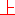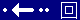Characteristics in 2D     Last updated on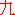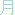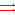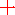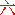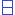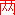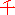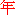, a full moon day

2 dimensional information flows ...; Approx. 37 characteristics are available to read; Also see: IC logical functions; Materials AND circuits; Transistor;

Channel length in µm vs. Threshold voltage in dV; Characteristics = Curve;

CMOS buffer propagation delay [in ns time OR in nm distance] vs. Output stage transistor size in nm;  Characteristics = Curve; Because it is a kind of Time vs. Voltage alike but the differences are Time vs. Voltage does not prompt structure of CMOS buffer; CMOS buffer propagation delay is caused by  its own internal structures of capacitive loads;

Control gate voltage VCG in V vs. Transistor current ID; Characteristics = Parallel straight lines in slope;   flash memory low Vt cell and high Vt cell at control gate voltage VR;

Date [14 days constraint] vs. Key generation rate;

Date [14 days constraint] vs. QBER;

Die per wafer: common characteristics are C temperature degree vs. mA,   impedance ohm vs. mA,   ms pulse width vs. W at peak power,   millisecond pulse width vs. W at power dissipation,   normalized voltage V vs. capacitance pF,   V vs. forward current' milli ampere mA,   voltage V vs. reverse current' mA, ... ;

Drain voltage in V vs. Drain current; Characteristics = Curve in linear region, line in slope in saturation region;

Drain voltage VDS in V vs. Drain current ID in m amp A; Characteristics = Curve in linear region, Straight line in saturation region;

Gate to Source voltage VGS in V vs. Normalized capacitance; Characteristics = Signal pattern;

Gate voltage in V vs. Drain current; Characteristics = Straight line to VT0 and then curve;

Input voltage in V vs. Output voltage V; Characteristics = Straight line to curve [cut-off point] to slope [forward active] to curve [cut-off point] to straight line; Typical; Also see: Ideal VTC in 1D;

Load capacitance in pF vs. Delay in ns; Characteristics = Line in slope; CMOS's slope > BiCMOS's slope;

Minimum-feature-size in micron in nm vs. Delay time in ns; Characteristics = Line in slope where typical gate delay and interconnect delay are indirectly propotional to each other in vector;

Monte Carlo probability density in decimal points vs. Propagation delay in ns; Characteristics = Non-consecutive dots in slope;

Negative substrate bias voltage VBB in V vs. Threshold voltage; Characteristics = Curve to represent cm-3;

nMOS Channel Width Wn in µm vs. Area x Delay Product; Characteristics = Curve to straight line in slope; where curve shows that global minima energy might exists;

nMOS Channel Width Wn in µm vs. Propagation delay in ns; Characteristics = Line in slope to curve to line in slope;

Output voltage Vout in V vs. Equivalent resistance R of CMOS; Characteristics = Curve; where Req, pMOS sine Req, nMOS is almost 1;

Power supply voltage VDD in V vs. Normalized delay in integer quantity; Vt and VDD's characteristics = Consecutive lines in slope and straight line;

Ratio t : h vs. FF, Fringing-field factor; Characteristics = Line in slope;

Ratio w : h vs. Capacitance in pF/cm; Characteristics = Line in slope;

Ratio w : h vs. FF; Characteristics = Line in slope;

Ratio w : l vs. FF; Characteristics = Line in slope;

Substrate bias in VSB voltage in V vs. Threshold voltage Vt; Characteristics = Line in slope;

Time in ms vs. Output current in mA of CMOS inverter circuit; Characteristics = Signal pattern;

Time in ms vs. Output voltage: 4V < Vout < 6.5V of CMOS inverter circuit; Characteristics = Fork signal pattern;

Time in ns vs. Amplitude V; Characteristics = Signal pattern;

Time in ns vs. Power supply current in mA; Characteristics = Pulse saw-tooth;

Time in ns vs. Power mW; Characteristics = Curve to straight line to curve to straight line to ... repeated steps upward;

Time in ns vs. Voltage V; Characteristics = Signal pattern;

Time in s [any given time] vs. Input voltage Vin; Characteristics = Signal pattern;

Time in s [any given time] vs. Output circuit current; Characteristics = Signal pattern; For basic system engineer: make you own circuit to reduce di / dt noise;

Time in s [any given time] vs. Output voltage Vout; Characteristics = Signal pattern;

Time in years vs. size in µm of integrated circuits; Characteristics = Curve;

Time in years vs. number of built-in transistors per chip; Characteristics = Straight line;

Trans-conductance Ratio kR in F vs. Switching threshold voltage; Characteristics = Curve;

Voltage V vs. Current I of SCR; Characteristics = Less than holding current IH, Curve; Higher than holding current IH, Line in slope;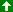Up